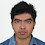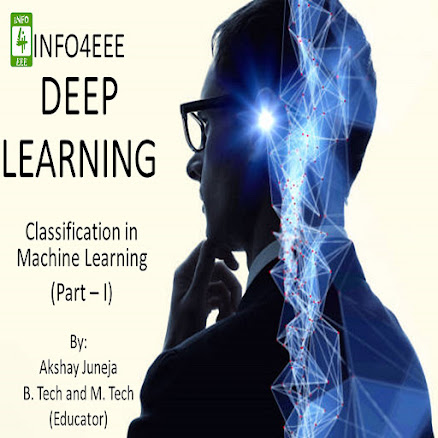Welcome back readers!! It has been a while since we talk via articles. Hope you all are doing great in your respective lives. So, let us continue from where we left in the previous article.

Classification in machine learning algorithms is either binary-class or multi-class. Binary classification is usually based on “YES” and “NO” outcomes. On the other hand, multi-class classification is used when there are more than two possible outcomes.

Various Classification-based techniques implemented in machine learning-based applications are as follows:

#### Neural Network

This section is an extended version of previously discussed topic – Artificial Intelligence. As we know, human brain consists of millions of neurons to transmit signals to and fro human brain. A neural network is an artificially designed brain which maps the output to the provided input, and weights are updated after each transfer of piece of information. Here we have 'm' inputs and 'n' outputs. Key points are as follows:

• Used with highly non-linear systems.
• Used when input data shows unexpected changes.
• Used when there is a need for constant updating in model.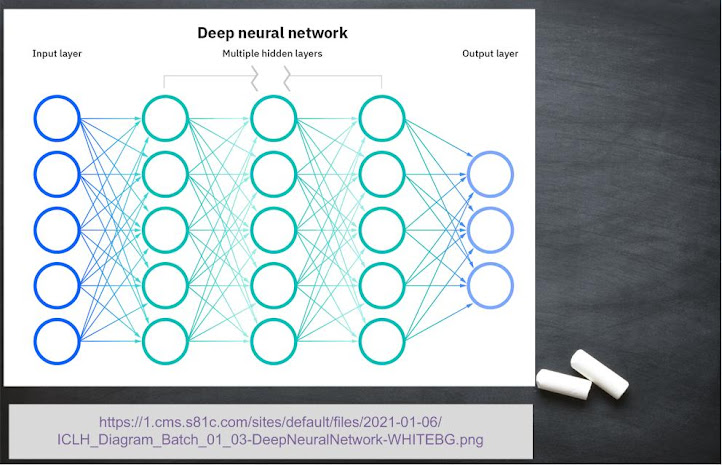#### Logistic Regression

Don’t go with the name. This is not a type of regression based machine learning technique. It is the basic type of classification-based technique. It is implemented in binary classes based problems. Key points are as follows:

• Used for linear separation of data using single boundary.
• It is the origin of different classification techniques.#### k-Nearest Neighbor

According to this algorithm, data of similar measures belong to same class. For instance, apple belongs to class of fruits along with banana and mango, not to a class of animals such as cat and dog. So, class of the input data is decided by the sample of data it is surrounded by. Key points are as follows:

• Used for data with large processing and storing memory.
• It is a slow process compared to neural network and logistic regression.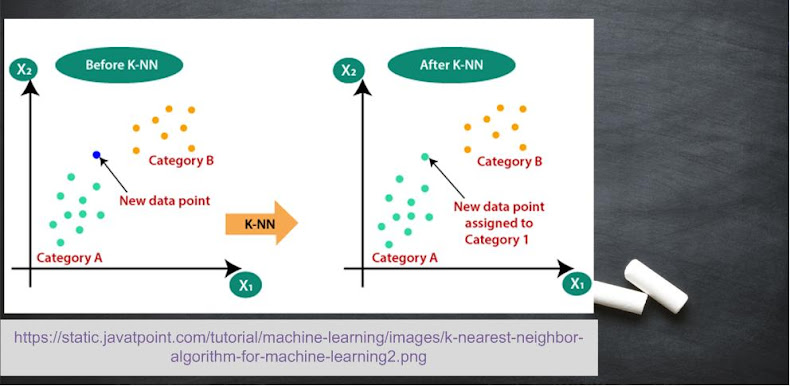#### Support Vector Machine

Unlike logistic regression, here you can go with the name 😄. A linear decision boundary (called hyperplane) is used to divide the data usually into two classes. Support vectors are the data elements of each class which are closest to the hyperplane. The task is to maximize the distance between these support vectors. For multiclass classification, support vector machine (SVM) is called error-correcting output code. Key points are as follows:

• Used for high-dimensional, non-linear classification.
• Used for better accuracy and interpretation.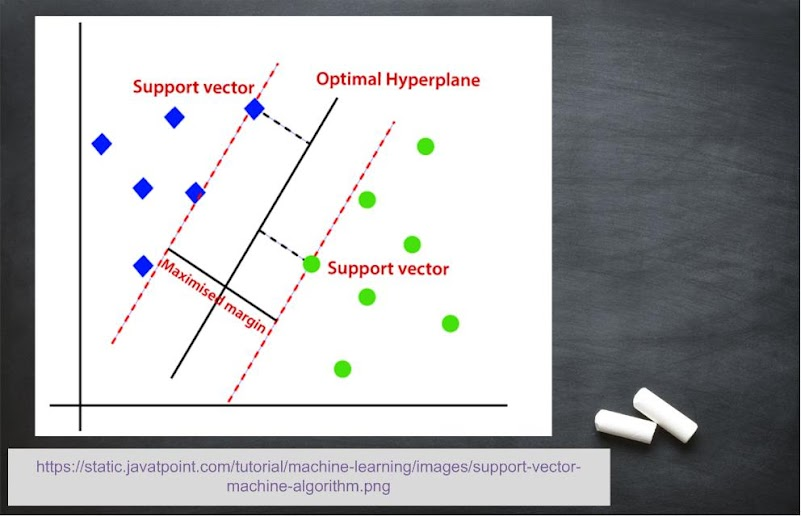Reference: Machine Learning with MATLAB (eBook)

Note: REMEMBER THAT THEORITICAL KNOWLEDGE IS EQUALLY IMPORTANT AS CODING. OUR TARGET IS TO MOVE STEP-BY-STEP UNIDIRECTIONALLY AND WE ARE CONCERNED WITH ALL TYPE OF READERS (BEGINNERS, INTERMEDIATES, and EXPERTS)

1.1.